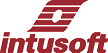Newsletter ListNewsletter Issue #78, November 2005

 Modeling Solar Cells

Most commercial solar panels are series/parallel combination of silicon photovoltaic, PV, p-n junctions. Each cell behaves like a silicon diode in parallel with a current generator. The magnitude of the current is proportional to the illumination. When a cell is exposed to the solar energy, photons with energy equal to the material bandgap (Eg) are converted into electrical energy. Photons with energy less than the bandgap do not contribute to the electrical energy. Photons with energy greater than the bandgap will waste energy greater than Eg. For Silicon, efficiency ranging from 10% to 20% is commercially available. Gallium Arsenide cells can be made with nearly double the efficiency of Silicon; however, GaAs is a more difficult (expensive) technology, mainly because of the brittle nature of the crystal. If multiple bandgap materials are used, the efficiency can grow beyond 50%. Very little has been done in recent years to extend photovoltaic (PV) device efficiency. Currently, DARPA (The Defense Advanced Research Projects Agency) is soliciting proposals to do just that.

 In This Issue 1 1 Economics of PV generation 3 4 Peak Power 5 Modeling Lithium-Ion Batteries 6 PV Lithium-Ion Battery chargers 9 Poly Crystalline Solar Panel 11 MPPT Implementation 14 Analog Multipliers 17 Phase Detector 18 Calculate Wire Length 19 New Transient Options
 Economics of PV generation
 The Solar Cell SPICE Model

For a simulator, the SPICE silicon diode coupled with a photocurrent generator and ohmic resistance does the trick. The model shown in Figure 1 shows how it’s done.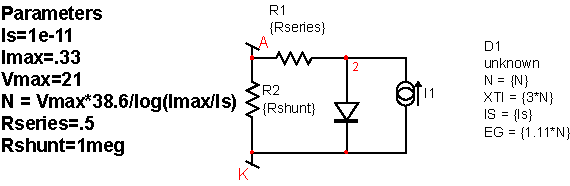Figure 1: SPICE solar cell model, scaled for series cells.

It’s assumed that solar cells are connected in series to achieve the desired open circuit voltage. Rather than connecting many diodes in series, it is convenient to scale the SPICE diode model. The diode model parameters that have been scaled are:

N, emission coefficient
EG, energy gap
XTI, temperature coefficient of Is
Is, saturation current.

The latter 3 parameters must be scaled to get the proper temperature coefficient. This model is correct if each cell is identical and has the same incident solar energy. If a shadow is cast, the cells with lower output current will determine the overall current. This is somewhat mitigated by connecting an external diode in reverse across each cell. Then if one cell has no output, the panel voltage will be reduced by the loss of voltage in the affected cell plus the drop in the auxiliary diode. For small panels, shadows and cell-to-cell variations shouldn’t make much difference, and the model simplification is justified. If, on the other hand you need to account for variability in illumination, you should replicate as many cells as are required.

You can calculate Is, N, Rseries and Rshunt based on test data for a single cell. Then the model can be extended to account for the number of cell in series to get Vmax. The diode model parameters are calculated based on Is, Vmax and Imax. The time constant created by the junction capacitance and the equivalent series resistance is short compared to the signals appearing at the terminals, therefore, the added model complexity to model the diode dynamics is unwarranted. It should be noted that a very large filter capacitor must be placed across the PV array if a switch mode power supply is used. This is so the switching cycles are averaged, and the filter capacitor is many orders of magnitude larger than the junction capacitance.

TOP

 Peak Power

There is a unique operating point for which maximum power is delivered. Figure 2 illustrates this for a typical mono-crystalline solar panel.Figure 2: Peak power is delivered at 83% of the open circuit output voltage.

The peak power operating point will vary with temperature and solar illumination. Happily, the high temperature case occurs when solar illumination is also high. That’s because it’s warmest on a sunny day. A rough estimate of the effect is made by assuming that about 50% of the incident solar illumination results in heating of the solar panel. Then the power density is .5*I*1e-4 watts per cm2 where I is the incident solar illumination in watts per m2. We know from thermal properties that in various semiconductors, heat transport raises temperature by about 300 Deg C. per watt/cm2. Moreover, the ambient temperature on a warm day is about 35 Deg. C and 20 Deg C. on a cooler day in a warm climate (assume .4*I). An equation can be written approximating the ambient temperature (Ta) as a function of solar illumination as:

Ta=10+.025*I

The temperature rise, Tr, is:

Tr=.015*I

And finally the solar cell temperature, Tc, is:

Tc = 10+.04*I

This is a very approximate calculation, but it allows a more aggressive design because the lower efficiency at high temperature is mitigated by the coincidently higher solar illumination.

Historically, solar panels have been expensive, such that obtaining maximum power has been an economic goal governing design of the power controllers. That is still the case today, and in the foreseeable future, for medium to high power PV arrays.

For low to medium power, a simplified approach can be used that connects the PV array directly to a battery. That approach sacrifices some solar energy, but has no power conversion loss, and no auxiliary housekeeping power loss.

As the power level increases, improved efficiency can be achieved with peak power tracking. The increased efficiency results in fewer solar cells for a given power requirement. That becomes a significant cost factor for arrays operating above 100 watts.

Tracking can be accomplished open-loop using the PV array temperature, or closed-loop using a peak power tracking control algorithm. These methods will be compared using a low power PV array to charge a Lithium-Ion battery, and a 10 watt array to charge a lead-acid battery. To make the comparison, a Lithium-Ion battery model is needed.

TOP

 Modeling Lithium-Ion Batteries

In the paper by Suleiman Abu-Sharkh and Dennis Doerffel , a battery model is described for a high power Lithium-Ion battery. An IsSpice4 model was made using the techniques and data described, and it was scaled down for use with a C cell (1.4 amp-hr, 3.6 volt). Figure 3 shows how the IsSpice4 model is implemented. Diodes D1 and D2 replace the zener diode in the Abu-Sharkh model. The nonlinear resistor, R01, is implemented using diode D3 and controlled current source B6. The scaled model tends to agree with various manufacturers published data.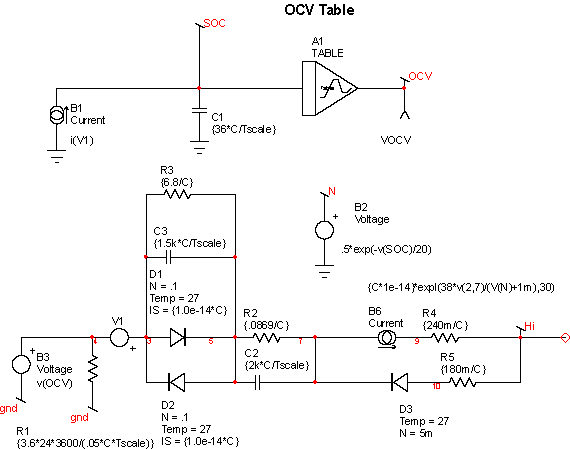Figure 3: The IsSpice4 model of a Lithium-Ion battery.

Batteries hold their charge for many hours, making a SPICE simulation impossible over the entire charge-discharge cycle when other circuit operations need to be simulated. This temporal mismatch is resolved by scaling the battery time by a factor of 3600, changing hours into seconds. Time constants on the order of seconds are long enough to appear to be steady state for surrounding circuitry, and are short enough to run simulations without running out of memory.

Batteries are characterized by their state of charge (SOC). The combination of B1 and C1 in Figure 3, captures the SOC. The batteries open circuit voltage, OCV, is taken from a table whose values come from test data. Parameters that define the specific model are:

C, battery capacity in ampere-hours
Tscale = 3600, time compression
SOC=100, the initial state of charge

 PV Lithium-Ion Battery chargers

Two battery charging techniques will be compared

1. Direct connection
2. Maximum peak power tracking, MPPT

In the first case, the number of series cells is varied so the “best” combination is chosen to work over the expected operating temperature range. Figure 4 shows the schematic, and resulting data is shown in Figure 5. The charger works best when the peak power voltage exceeds the battery voltage. If the maximum cell temperature is 60 Deg C. then 10 cells are required. The parameter calculation uses the cell-temperature estimate developed earlier, and adds the concept of a normalized incident radiation, sol, where sol=1 for AM1.5 conditions. Notice the voltage clamp using D3 and V5. Lithium-Ion batteries must not be overcharged for safety concerns. The voltage clamp, along with short circuit protection is internal to consumer cells. OEM cells require the manufacturer to provide safety features that include:

Over voltage limit, 4.2 volt
Under voltage limit, 3 volt
Short circuit fuseFigure 4: Charging with 10 IXYS XOD17-48B cells in series.Figure 5: Battery fully charged on a bright summer day, and less than 50% in winter.

This technique wastes incident solar energy and requires mode solar cells than a peak power tracking solution would require.

The peak power vs. solar illumination is plotted in Figure 6. Peak power tracking can be simulated using a behavioral element to supply constant power to the Lithium-Ion battery as shown in Figure 7.Figure 6: Solar array characteristic vs. incident solar radiation.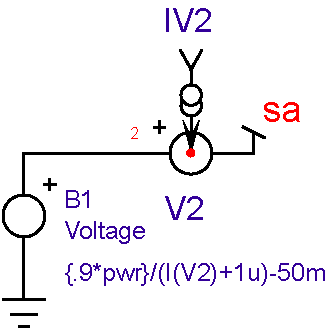Figure 7: A simulated source includes efficiency and housekeeping power estimates.

Plugging in the power values into the array simulation results in the data shown in Figure 8. Unfortunately, the power loss from housekeeping power and converter efficiency wipes out the MPPT gain. So, it’s unnecessary to proceed further with the design because the simpler direct charge method produces superior results.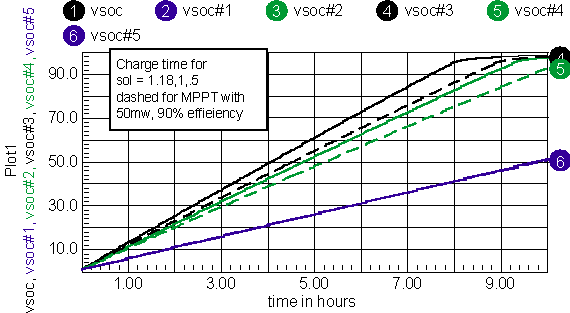Figure 8: Charge times with MPPT are dashed curves.

TOP
 Poly Crystalline Solar Panel

Poly crystalline panels use a less efficient and lower cost technology. This technology is widely used in lower power modules. The cost for a 10-watt module runs about \$100. These small panels are used widely in undeveloped regions to operate electronic devices, such as TV’s or PC’s, for a few hours a day. The arrays are built to charge a Lead-Acid gel type battery. This battery can be “over-charged” to equalize the SOC in the series cells. It is necessary to keep the over-charge voltage low enough to prevent formation of Hydrogen gas. The poly crystalline PV array isn’t accurately modeled using the mono-crystalline model, as shown in Figure 9.

 Figure 9: The mono-crystalline model has errors at the endpoints for a Shell ST10 module.

Several additions to the model are needed for the Poly crystalline array. First, in 1996, Zekry, et. al. , pointed out that lateral resistance in the array could be modeled by using a distributed model, that is, parallel diodes and photocurrent generators connected by resistors. If 4 such sections are used, the problem can be reduced to 2 sections by recognizing symmetry between the 2 anode contacts. Adding this affect gets a fit at higher array voltages (high diode current). Next, the generation-recombination current needs to be included. This was done by adding a parallel diode with 2*N emission coefficient. The extra diodes series resistance and saturation current were used as free parameters to make the best fit to the data. Figure 10 shows how the model compares to the published data at 20 and 60 Deg C for the Shell ST10 module. Figure 11 shows the model schematic.

The solar array peak power data are shown in Figure 10.Figure 10: The final model compared with published data for the Shell ST10 module.Figure 11: A poly crystalline PV Solar Array Model.

Next, the steps in the previous Lithium-Ion battery charger can be repeated using the poly crystalline model and a Lead-Acid battery. First, the solar array power vs. illumination curves is used to determine the peak power tracking points vs. illumination. These data are shown in Figure 12.Figure 12: Peak power varies with solar input and temperature.

Fortunately, we already have a Lead-Acid battery model, so it’s only necessary to connect the array directly to the battery to get the charge times. Then, using the previously computed peak power operating points, the charge times can be calculated using a constant power source to charge the battery. The results are shown in Figure 13.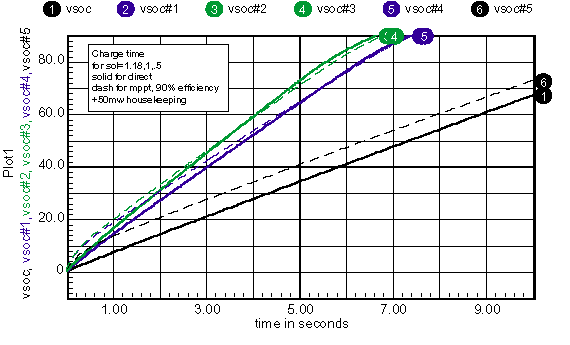Figure 13: Charge time with MPPT is comparable to direct charging except for low solar illumination.

The only substantial benefit for MPPT tracking is to increase the SOC from 54% to 60% in the winter. That would not seem to justify the increased complexity in this application. So, when would you use peak power point tracking? Evidently the overhead in terms of power and circuit complexity must justify the inclusion of MPPT. For battery chargers, once you get to a 100% state of charge, then nothing you do will improve the situation. That means MPPT is only useful when the solar radiation is low, or varies a lot in cloudy climate. Even then, it’s only a 10% or so improvement, which is easily gained be increasing the size of the solar panel. On the other hand, if the battery is oversized, it will never fully charge and the maximum energy can be recovered. That’s equivalent to returning energy back to the power grid. Moreover, when energy is returned to the power grid, there must be a switching power supply so that its inefficiency doesn’t penalize the MPPT. These larger systems won’t notice the housekeeping power loss. Saving 10% on a \$50,000 installation is certainly worth adding MPPT, even at the cost of a microprocessor.

TOP

 MPPT Implementation

The peak power operating point can be tracked using a controller, or by using an estimate based on array temperature. In either case, the controlled state variable must be chosen. Normally the output current would be selected. But the output current is proportional to power and there will be two possible operating points.

Therefore, controlling either power or output current directly results in a small signal gain reversal at the maximum power point, so that the control loop would be statically unstable. That’s a common problem encountered in control systems. For example, an airplane (or car) that has its center of gravity too far to the rear will try to fly (or drive) backwards if there is an angular disturbance. The problem is usually solved by controlling a different parameter in the “inner” control loop. Here, the array voltage can be controlled without any static instability, so the array voltage becomes the output of the inner or high-speed control loop. For an open-loop controller it’s necessary to estimate the array voltage vs. temperature. There will be some uncertainty in the estimate because of ageing and a slight dependence of solar illumination.

To eliminate this error, the peak power point can be measured and the control signal modified to settle at the peak. Measuring the peak power point requires the introduction of some kind of disturbance. The approach taken by most commercial operations is to use a microprocessor unit (MPU) that perturbs the operating point, and corrects the output based on which side of the maximum the current set point is detected. Another analog approach (the mpu could do this also) is to introduce a dithering frequency and designing a “linear” control law that seeks the maximum power point. The microprocessor-based approach can use the “linear” control laws, or it can be based on nonlinear control techniques, such as fuzzy logic or even neural networks. The advantage of using the “linear” approach lies in applying the well-established control system theory to describe the loop dynamics.

To find a maximum of a function, its derivative can be taken and the maximum occurs when the derivative is zero. Differentiating P=IV, give the following:Notice that dv and di can be considered “small” signal parameters. So, if a relatively low frequency AC signal is introduced into the system, then di and dv can be extracted using a band-pass filter. Then the value at the dither frequency can be evaluated by demodulating the result. Now, here’s where simulation can be used to design the control system. Rather than writing the equations, the simulator can be used to evaluate the control law and set scale factors. Figure 14 shows how that’s done for the default array. First, the previously discussed solar panel model is used in X3, then X1 models a buck regulator operating in continuous conduction mode. Nodes dv and di are the dither signals extracted as though they are the only AC signals present. Later-on the PWM switching signal must be filtered. B2 is the control law for making the array voltage equal to the control signal. Finally, Vc is metered to see how it varies as the array voltage is swept using the control signal, Vs. Vs includes the dither signal from V2. Figure 15 shows the transient simulation results. Next, the signal from Vc is used for control and a step change in load is introduced to check on loop dynamics. This was accomplished by changing the B2 expression to
V=v(10)*.01+v(vc)*10+{12/17}Figure 14: System level simulation.Figure 15: The simulated control signal goes through zero at maximum power.Figure 16: Power tracking works when V6 steps down 20ms into the simulation.

Having made an acceptable control law, the circuit implementation is needed. Three multiplications are required. That’s fairly expensive if general purpose analog multipliers are used; it’s not so bad for a microprocessor, but the sample rate needs to be high compared with the dither frequency. Continuing from an analog design perspective, the I*dv and V*di products are no more than variable gain circuits.

TOP

 Analog Multipliers

Sometime in the 60s an unknown author described how to do this economically using a field effect transistor. Both JFETs and MOSFETs operated in the “linear” region (that’s physics talk for the engineers saturated region) follow the equation shown below for the grounded source configuration: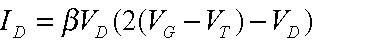For MOSFETS, you set,where W is the channel width, L is the length, and BETA and Kp are gain parameters. Both MOSFETS and JFETs work with slightly negative Drain-Source voltages (less than a diode drop). Now if you connect a large valued resistor between the drain and gate and an equal valued resistor to a control signal, then,Substituting back into the first equation,And the conductance is then proportional to the control voltage. Placing the FET at the input to an op-amp has the effect of making a 2-quadrant multiplier shown in Figure 17.Figure 17: A simple inexpensive multiplier.

As the FET gate voltage exceeds threshold, the FET becomes a resistor. The threshold voltage varies considerably with time and temperature, so you should keep away from the threshold by a volt or so. As the voltage is increased further, the resistance decreases. In the limit, the resistance can’t decrease below the bulk resistance. These upper and lower boundaries limit the useful range of this gain control technique to something on the order of 10:1. For a larger dynamic range, diodes can be used. The circuit is more complex because the diode’s voltage offset must be cancelled by using a pair of diodes. Figure 18 shows the basic idea.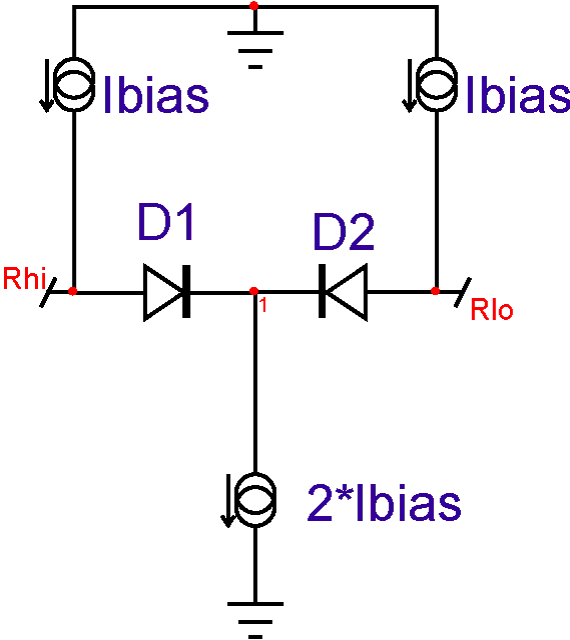Figure 18: The conductance between Rhi and Rlo is proportional to Ibias.

In forward conduction, the diode equation iswhere

q= charge of an electron=1.60218E-019 coulombs
N= emission coefficient
K= Boltzmans constant = 1.38066E-023 (coulomb volt) / (kelvin)
T= temperature in deg. kelvin.
Io is a device parameter

Solving for conductance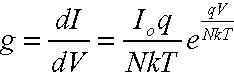Then substituting I back into the conductance equationThis result holds over a remarkable range of current and is the basis for nearly all IC multiplier circuits.

Figure 19 shows an IC implementation that can also be used with transistor arrays. It is limited at high I by the bulk resistance and at the low end by leakage current. You can easily coax an accurate result over a 3-decade range with this circuit. The diode-connected transistors (Q5 and Q6) reduce the effect of bulk collector resistance because of the transistor action. That extends the small-signal performance range by the transistor current gain. The gain control current is supplied to the Igain node of Q3. Current mirrors supply Igain to each of the diode connected transistors, and a balancing current to their emitters. Rhi and rlo are the variable resistor terminals that must be biased between the Vcc-Vee power rails.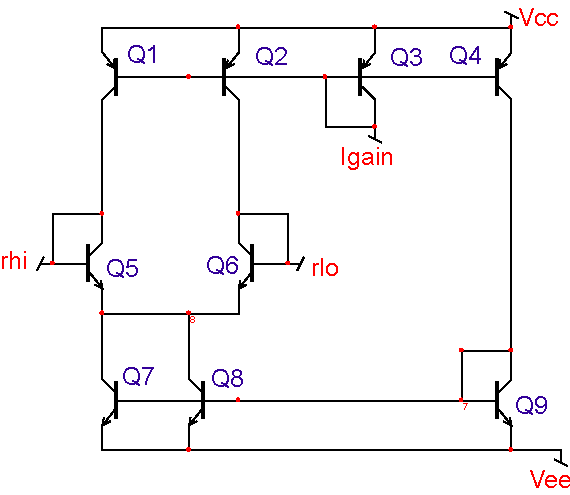Figure 19: Current mirrors bias the diode connected transistors, Q5 and Q6.

The FET resistance modulation scheme will be used for the low cost analog peak power tracker.

TOP

 Phase Detector

The final product, where dp is multiplied by v(10), can actually be considered to be a phase detector. That’s accomplished by using the polarity of v(10), which is used to select the positive or negative dp result. Moreover, dp can also be limited, and the result is accomplished using an exclusive or gate. Figure 20 shows the result, comparing the xor with a multiplier for sol=.5. The schematic for this test case is in the drawing file named MPPT_MUL1.DWG. The PWM control was set to voltage mode and the array voltage was swept from 12 volts to 21 volts from about 20ms to 50ms. A 1 kHz dither signal was inserted in the control loop such that the AC array voltage was the same for all DC sweep values. Notice that the ripple in power minimizes at the peak power point.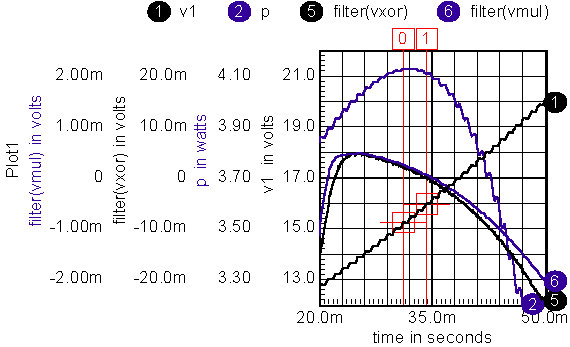Figure 20: XOR circuit replaces multiplier.

The raw products were filtered in IntuScope using 5th-order Bessel filters, Delay=3m. Therefore, you must look at the data 3ms earlier to get the correct steady state values. The cursors are set at the zero crossing and 3ms prior to the zero crossing. Both the xor and multiplier produce about the same zero crossing. The peak power point is correctly detected at the zero crossing for both cases. Power stays within 2% of the peak over a 2.5-volt range of array voltage. The array voltage should be easily estimated within that band by accounting for solar cell temperature. That suggests the simpler open-loop MPPT would be acceptable for anything but the highest cost systems. Temperature can be measured using a forward biased silicon diode attached to the array. The array voltage for MPPT is then proportional to the diode voltage.

  S.M.Zee, Physics of Semiconductor Devices, 2nd edition, John Wiley & Sons,1981,pg 793.  S.M.Zee, Physics of Semiconductor Devices, 2nd edition, John Wiley & Sons,1981,pg 806.  Suleiman Abu-Sharkh and Dennis Doerffel , Rapid test and non-linear model characterization of solid-state lithium-ion batteries, First International Symposium on LLIBTA in Honolulu, Hawaii, June 2005.  Abdelhalin Zekry and Abdulhameed Yousef, A Distributed SPICE-Model of a Solar Cell, IEEE Transactions on Electron Devices, Vol. 43, May 1996, pg 691.

TOP
 Calculate Wire Length

To determine the “wire length” of each winding in Magnetics Designer you can do the following:

2. Check “for each winding,” “For Inductors,” and “For Transformers”
3. In the description field type “Winding Length”
4. In the button label field type “WireLength”
5. Press the “>>next>>” button
6. In the equation section add the following:

WireLength = 0
sum_build = 0
shape == wShape ? 3.14159 : 4
for( winding = 0 ; winding < Nmax ; winding = winding+1 )
WireLength(winding) = N(winding)*shape*(ID + 2*sum_build + build(winding) - tw(winding))
sum_build = sum_build + build(winding)

7. Press the “>>next>>” button
Note: If you want to make this value available for future designs you need to add what is shown to the bottom of the user.equ file.
8. Press the “>>finish>>” button

At the bottom of each winding you should see the Winding Length given in cm. Also the values will be shown in the summary report.

TOP

 New Transient Options

Intusoft has implemented two new powerful features for IsSpice4 Transient Analysis. In the new 8.x.11 Build 2641, new convergence options have been added to improve the accuracy and speed of transient analysis.
The first option is called VSECVMAX. If this option is set, the value is used as an upper limit for applying the VSECTOL convergence algorithm during a transient analysis. Setting this option prevents the VSECTOL algorithm from getting trapped into divide-by-zero situations. A typical value is 1E4. Note that this option is only valid when VSECTOL is set. As an example:

.options vsectol = 1u vsecvmax=1e4

The second new feature is a novel way of using ITL4 in transient analysis. Recall that ITL4 is an option commonly used in SPICE-based simulators to control the number of iterations in each transient time point calculation. Up to now, ITL4 has been set by users to a large value (typically 100-500) in order to avoid the dreaded “time step too small” error in transient analysis. The problem with this approach is that it may result in making transient analysis unnecessarily longer than needed for most time points. Since the number is fixed, the simulator will scale back the time step after it has gone through that many iterations at every time point. This results in a long simulation run time.

In Build 2641, we are introducing a new dynamic control for ITL4. Simply set ITL4=0 in an options statement and the simulator will automatically determine the maximum number of iterations to try for each transient time point, before scaling back the time step value. At the end of the simulation you can check the “maximum transient iterations” to see what was the maximum number of iterations that the simulator took to complete the simulation. This figure is usually well below the value that you might have set for ITL4.

As an example, consider the IR1150 TestBridge drawing. Running a 20ms transient simulation with ITL4=200 verses ITL4=0 results in the following statistics at the end of the run:As you can see there is a big improvement in both the total number of iterations and the total run time when using the new algorithm. We used an AMD Athlon64 3200 with 1G of ram in running these simulations.

We request that you try this new approach in simulating hard-to-converge and long transient analysis designs. Please report your findings to us (rmktg@intusoft.com). This will in turn help us fine tune this algorithm and make it perform even better in future releases.